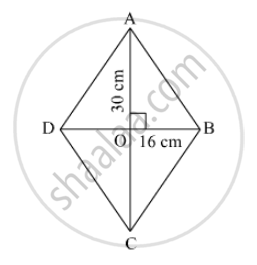Advertisement Remove all ads

# If Length of a Diagonal of a Rhombus is 30 Cm and Its Area is 240 Sq Cm, Find Its Perimeter. - Mathematics

Sum

If length of a diagonal of a rhombus is 30 cm and its area is 240 sq cm, find its perimeter.

Advertisement Remove all ads

#### SolutionLet the other diagonal be d cm
Area of a rhombus = 1/2 (product of diagnols)

⇒ 240 = 1/2 xx (30 x d)

⇒ d = (240 xx 2)/30 = 16

AC = 30 cm
DB = 16 cm
Diagonals of a rhombus bisect at right angles.
In Δ AOB,

AO² + OB² = AB²

⇒ 15² + 8² = AB²

⇒ AB² = 225 + 64 = 289

⇒ AB = √289 = 17 cm

Thus, the side of the rhombus = 17 cm
Perimeter = 4 xx 17 = 68 cm

Is there an error in this question or solution?
Advertisement Remove all ads

#### APPEARS IN

Balbharati Mathematics 8th Standard Maharashtra State Board
Chapter 15 Area
Practice Set 15.2 | Q 4 | Page 97
Advertisement Remove all ads
Advertisement Remove all ads
Share
Notifications

View all notifications

Forgot password?